# Lense Shift

## Canvas subdivision for non parallel projections

Take a canvas with the following properties: `W`x`H` with a fov defined as `F`

Example: 960x540 (16/9) @ 70 FoV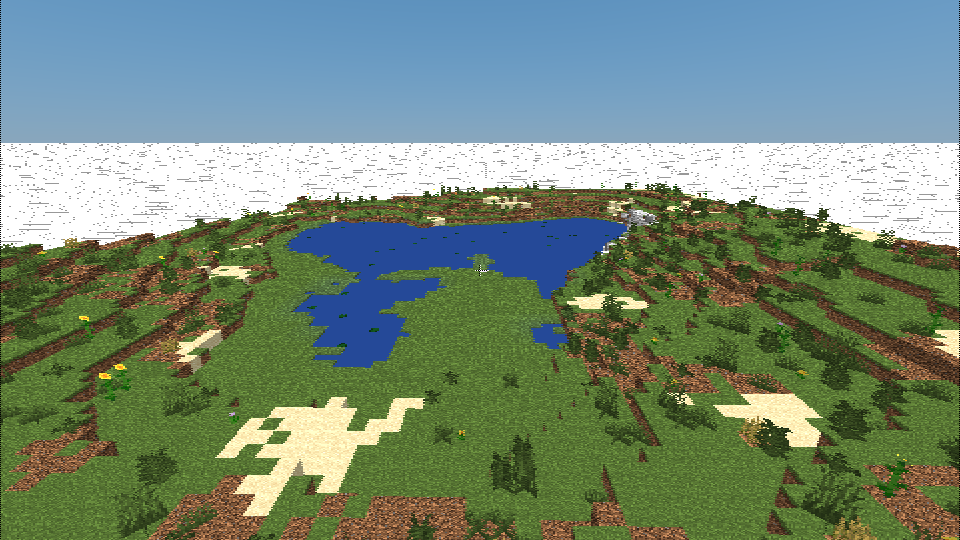### Subdivision by half

To calculate the required lense shift values to render two smaller canvases (side-by-side) with a [`W`/2 x `H`] = [`w` x `h`] size, given it’s relation to canvas height, only the ratio between the `w` & `h` of the sub-canvas is required to be calulated

`x = +/- 1/2 * w/h`

No FoV change required.

This gives us the following two lense shift combinations:

Examples at 480x540 @ 70 FoV

[-0.5w/h,0] [0.5w/h,0]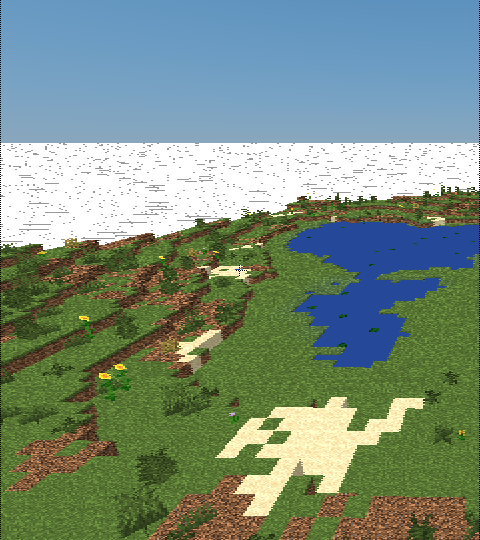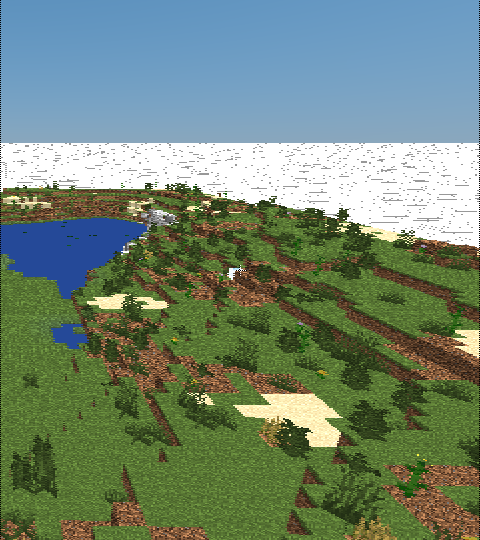E.G. For the provided subdivisions the offsets would be [+-0.444444444,0].

### Subdivision by quarters

To calculate the required lense shift values to render four smaller canvases with a [`W`/2 x `H`/2] = [`w` x `h`] size with a `F`/2 = `f` FoV, given it’s relation to canvas height, only the ratio between the `w` & `h` of the sub-canvas is required to be calulated:

`x = +/- 1/2 * w/h`

&

`y = +/- 1/2`

This gives us the following lense shift combinations:

Examples at 480x270 @ 35 FoV

[-0.5w/h,+1/2] [0.5w/h,+1/2]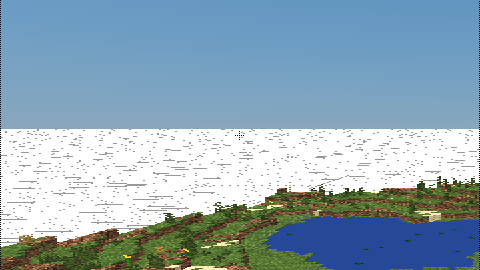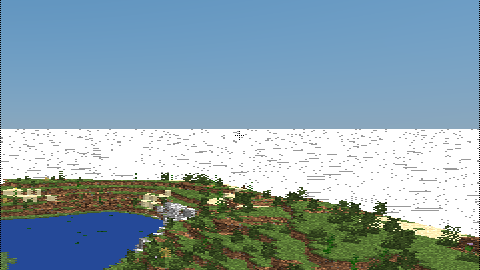[-0.5w/h,-1/2] [0.5w/h,-1/2]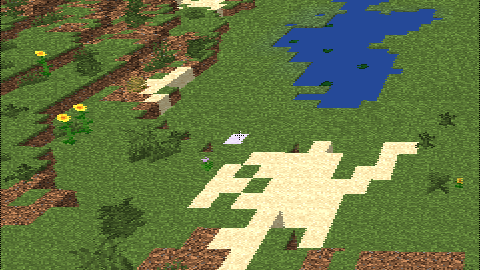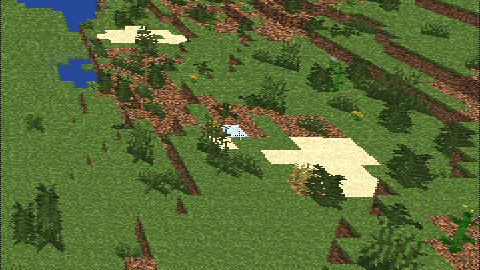E.G. For the provided subdivisions the offsets would be [+-0.888888889, +-0.5].

### Ninth part subdivision

To calculate the required lense shift values to render nine smaller canvases with a [`W`/3 x `H`/3] = [`w` x `h`] size with a `F`/3? = `f` FoV, given it’s relation to canvas height, only the ratio between the `w` & `h` of the sub-canvas is required to be calulated:

WIP

### Sixteenth part subdivision

To calculate the required lense shift values to render sixteen smaller canvases with a [`W`/4 x `H`/4] = [`w` x `h`] size with a `F`/4? = `f` FoV, given it’s relation to canvas height, only the ratio between the `w` & `h` of the sub-canvas is required to be calulated:

WIP